Next: The Bernoulli model Up: Naive Bayes text classification Previous: Naive Bayes text classification   Contents   Index

## Relation to multinomial unigram language model

The multinomial NB model is formally identical to the multinomial unigram language model (Section 12.2.1 , page 12.2.1 ). In particular, Equation 113 is a special case of Equation 104 from page 12.2.1 , which we repeat here for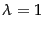: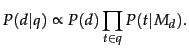(120)

The document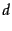in text classification (Equation 113) takes the role of the query in language modeling (Equation 120) and the classes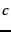in text classification take the role of the documentsin language modeling. We used Equation 120 to rank documents according to the probability that they are relevant to the query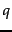. In NB classification, we are usually only interested in the top-ranked class.

We also used MLE estimates in Section 12.2.2 (page) and encountered the problem of zero estimates owing to sparse data (page 12.2.2 ); but instead of add-one smoothing, we used a mixture of two distributions to address the problem there. Add-one smoothing is closely related to add-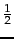smoothing in Section 11.3.4 (page).

Exercises.

• Why is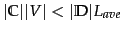in Table 13.2 expected to hold for most text collections ?Next: The Bernoulli model Up: Naive Bayes text classification Previous: Naive Bayes text classification   Contents   Index